# Part 1: Indices | Beginner’s Guide to Year 8 Maths

Indices in Year 8 are a crucial foundation for mastering higher levels of algebra. Here is our guide to ensuring your success with some tips that you should check out before going on to Year 9.How well do you understand Year 8 indices? Well, whether you’re a beginner or just brushing up, this Guide will get you up to speed so you can ace Year 8 Maths.

## The NSW Syllabus Outcomes we’ll explore

• Investigate index notation and represent whole numbers as products of powers of prime numbers (ACMNA149)
1. Express a number as a product of its prime factors, using index notation where appropriate
• Use index notation with numbers to establish the index laws with positive-integer indices and the zero index (ACMNA182)
1. Use index laws to simplify expressions with numerical bases
• Apply index laws to numerical expressions with integer indices
1. Establish the meaning of negative indices with numerical bases, e.g. by patterns
2. Evaluate numerical expressions involving a negative index by first rewriting with a positive index
• Define rational and irrational numbers and perform operations with surds and fractional indices (ACMNA264)
1. Apply index laws to demonstrate the appropriateness of the definition of the fractional index representing the square roots
2. Apply index laws to demonstrate the appropriateness of the following definitions for fractional indices: $$x^{\frac{1}{n}} = \sqrt[n]{x}, x^{\frac{m}{n}} = \sqrt[n]{x^m}$$

## What we’re going to discuss

We will be covering the following topics:

## Assumed knowledge

Students should be able to recognise and evaluate basic indices (e.g. $$2^3 = 2 \times 2 \times 2 = 8$$),  as well as the meaning and application of square and cubes roots (e.g. $$\sqrt{64} = 8$$).

Students should also be familiar with the order of operations (i.e. BODMAS: Bracket, Order, Division/Multiplication, Addition/Subtraction).

## Want to test your knowledge of Indices before we start?## Factor Trees

Factor trees can be used to express a number as a product of its prime factors.

Consider the factor tree for the number $$72$$.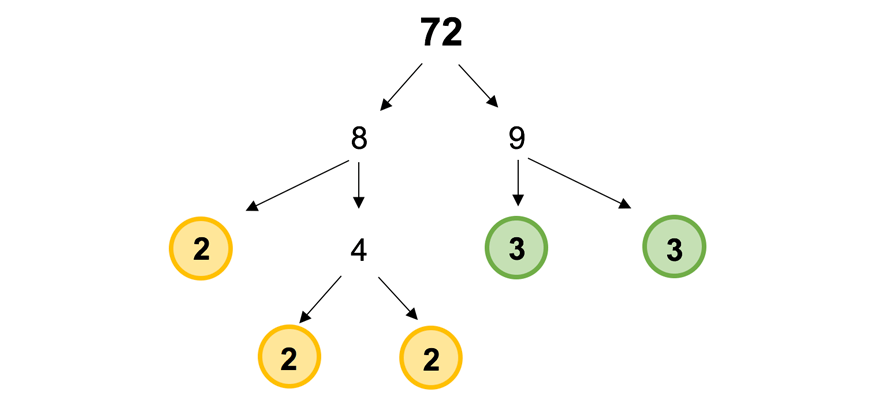Note how we keep dividing each factor into smaller factors until we are left with only prime numbers.

Although there are multiple different ways to construct this factor tree (e.g. we could have started with $$6$$ and $$12$$), by following this rule, we will ultimately get to the same result.

Reading the factors at the ends of the tree, we can now express $$72$$ as a product of its prime factors:

$$72 = \color{goldenrod}{2 \times 2 \times 2} \times \color{green}{3 \times 3}$$

This expansion can be re-written more simply using indices:

$$72 = \color{goldenrod}{2^3} \times \color{green}{3^2}$$

Factor trees are a useful technique to help us systematically approach harder questions such as:

• Finding the highest common factor (HCF)
• Determining whether a number is a perfect square or cube

### Example: Finding the highest common factor

1. Consider the numbers $$36$$ and $$90$$. By expressing both numbers as a product of prime factors, find their highest common factor.

Recall that the highest common factor refers to the biggest number that both $$36$$ and $$90$$ can be divided by.

Solution

First we must complete the factor trees for both $$36$$ and $$90$$.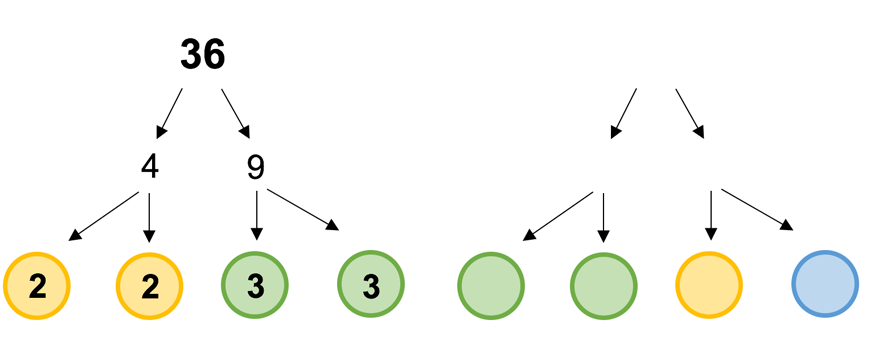Using the factor trees, we can now re-write $$36$$ and $$90$$ as a product of their prime factors:

\begin{align*}
36 &= \color{goldenrod}{2^2} \times \color{green}{3^2}\\
90 &= \color{goldenrod}{2} \times \color{green}{3^2} \times \color{blue}{5}
\end{align*}

Since the HCF is a factor of both $$36$$ and $$90$$, we know that it must be possible to express the HCF as a product of these prime factors. Considering each prime factor individually:

• The factor $$\color{goldenrod}{2}$$ occurs a maximum of one time across both $$36$$ and $$90$$. Hence, it only occurs once in the HCF (i.e. $$2^1$$)
• The factor $$\color{green}{3}$$ occurs twice in both $$36$$ and $$90$$. Hence must be a factor of the HCF.
• The factor $$\color{blue}{5}$$ only occurs in $$90$$, but not $$36$$. Hence it is not a factor of the HCF.

This tells us that the HCF must be:

\begin{align*}
HCF &= \color{goldenrod}{2^1} \times \color{green}{3^2}\\
HCF &= 2 \times 9\\
HCF &= 18
\end{align*}

## Index Laws

Index laws allow us to simplify an expression involving multiple indices.

### Powers of the Same Base

The table below summarises the basic index laws.

Note that, these laws only apply for powers on the same base.

 Multiplication $$x^m \times x^n = x^{m+n}$$ Division $$\frac{x^m}{x^n} = x^{m-n}$$ Powers of powers $$(x^m)^n = x^{m \times n}$$

When multiplying powers of the same base, we can simply add the powers together.

For example:

\begin{align*}
3^2 \times 3^3 &= 3^{2+3}\\
&= 3^5\\
&=243
\end{align*}

Similarly, if we divide powers of the same base, we can simply subtract the powers from each other.

For example:

\begin{align*}
\frac{5^7}{5^5} &= 5^{7=5}\\
&= 5^2 \\
&= 25
\end{align*}

When we take the power of a power we can simply multiply the powers together.

For example:

\begin{align*}
(10^2)^3 &= 10^{2 \times3}\\
&= 10^6
\end{align*}

### The Distributive Law

Powers of brackets can be expanded using the distributive law.

This property is shown below:

\begin{align*}
(x \times y)^n = x^n \times y^n
\end{align*}

For example, we can say:

\begin{align*}
(x \times 5)^2 &= 3^2 \times 5^2\\
&= 9 \times 25\\
&= 225
\end{align*}

This rule also applies to fractions, for example:

\begin{align*}
\big{(}\frac{4}{7}\big{)}^3 &= \frac{4^3}{7^3}\\
&= \frac{62}{343}
\end{align*}

Interestingly, we can even apply this rule to square and cube roots, as illustrated below.

The reasons for this will be explained in the section Fractional Indices.

\begin{align*}
\sqrt{9 \times 25} &= \sqrt{9} \times \sqrt{25} \\
&=3 \times 5\\
&= 15
\end{align*}

The distributive law also works in the opposite direction, when you have two numbers taken to the same power:

\begin{align*}
5^2 \times 2^2 &= (5 \times2)^2\\
&= 10^2 \\
&= 100
\end{align*}

### Common Mistakes

It is important to remember that the distributive law only applies for single terms (i.e. brackets containing only multiplication or division).

We cannot use the distributive law to expand brackets containing addition or subtraction

For example $$(3 + 6) \neq 3^2 + 6^2$$

### Example

1. Evaluate the following expression:

\begin{align*}
2^4 \times 3^4 \div 6^2 – (3+2)^2
\end{align*}

Solution

When approaching a complicated expression such as this one, we need to remember our order of operations from BODMAS (Bracket, Order, Division/Multiplication, Addition/Subtraction).

First, we must simplify the terms inside the brackets.

\begin{align*}
2^4 \times 3^4 \div 6^2 – \color{red}{(3 + 2)^2} = 2^4 \times 3^4 \div^2 – \color{red}{5^2}
\end{align*}

Note that, $$2^4$$ and $$3^4$$ have the same power. Using the distributive law, we can simplify this into a single expression.

Now that we have two power of base $$6$$, we can use our index laws to simplify this further.

\begin{align*}
\color{red}{6^4 \div 6^2} – 5^2 = \color{red}{6^2} – 5^2
\end{align*}

Evaluating both power terms, we get:
\begin{align*}
6^2 – 5^2 &= 36 – 25 \\
&= 11
\end{align*}

## Negative Indices

The following results are useful to remember when working with non-positive indices.

 Zero Index $$x^0 = 1$$ Negative Indices $$x^{-n} = \frac{1}{x^n}$$

Any number taken to the power of $$0$$ is equal to $$1$$.

For example:

\begin{align*}
2^0 &= 1\\
5^0 &= 1 \\
100^0 &= 1
\end{align*}

Taking a number to a negative power turns it into a fraction. To do this, we make the power positive and make it the denominator.

For example:

\begin{align*}
2^{-3} &= \frac{1}{2^3} \\
&= \frac{1}{8}
\end{align*}

The reason for these results can be illustrated using a table of indices.

Consider the following powers of $$2$$. Notice that we divide the number $$2$$ every time we decrease the power by $$1$$.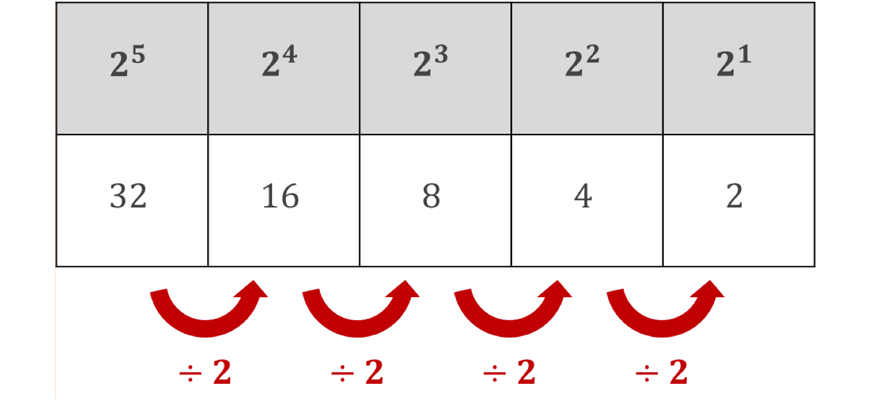By dividing two each time, we can extend this table.

Pay close attention to the zero index and the negative indices.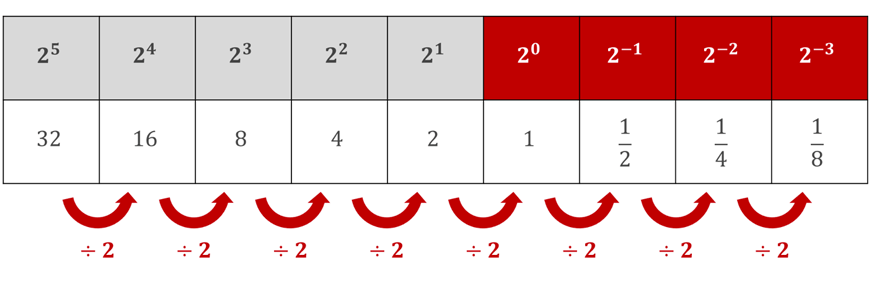By re-writing the denominators as powers of $$2$$, we can observe that the result $$x^{-n} = \frac{1}{x^n}$$ holds true.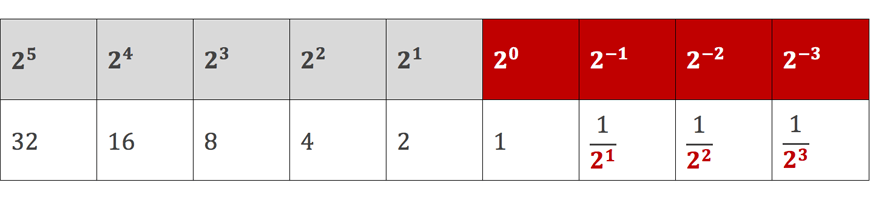### Example: The Zero Index

1. Evaluate the following expression:

\begin{align*}
13 \times 2^{-3} \div 3^{-5}
\end{align*}

Solution

Recall that any expression to the power of $$0$$ will equal to $$1$$.

Since there are no more indices, we can simply work out the value of the expression:

\begin{align*}
23 – 2(1) – 1 &= 23 – 2 – 1\\
&= 20
\end{align*}

### Example: Negative Indices

1. Express the following using only positive powers:

\begin{align*}
12 \times 2^{-3} \div 3^{-5}
\end{align*}

Solution

To start we can re-write all the negative indices as fractions using the result $$x^{-n} = \frac{1}{x^n}$$.

\begin{align*}
12 \times \color{goldenrod}{2^{-3}} \div \color{green}{3^{-5}} = 13 \times \color{goldenrod}{\frac{1}{2^3}} \div \color{green}{\frac{1}{3^5}}
\end{align*}

Since we are dividing by the fraction $$\frac{1}{3^5}$$, we can multiply by its reciprocal.

\begin{align*}
13 \times \frac{1}{2^3} \div \color{green}{\frac{1}{3^5}} = 13 \times \frac{1}{2^3} \div \color{green}{\frac{1}{3^5}}
\end{align*}

By multiplying together the numerators and denominators, we can simplify this expression.

\begin{align*}
13 \times \frac{1}{2^3} \times \frac{3^5}{1} &= \frac{12 \times 1 \times 3^5}{2^3 \times 1}\\
&= \frac{13 \times 3^5}{2^3}
\end{align*}

## Fractional Indices

Remember that square roots ($$\sqrt{x}$$) and cube roots ( $$\sqrt{x}$$) are simply the reverse operation to squaring or cubing a number.

For example:

Since $$7^2 = 49$$ then $$\sqrt{49} = 7$$.

Similarly, since $$4^3 = 64$$ then $$\sqrt{64} = 4$$.

We can express roots as fractional indices using the following results:

 Fractional indices $$x^{\frac{1}{n}} = \sqrt[n]{x}$$ $$x^{\frac{m}{n}} = \sqrt[m]{x^m}$$

This can be proved using the index laws we have already covered.

For example,

consider $$\big{(} 15^{\frac{1}{3}} \big{)}^3$$.

Using the power of powers law, we know that $$\big{(} 15^{\frac{1}{3}} \big{)}^3 = 15^{\frac{1}{3} \times 3} = 15^1 = 15$$.

Since we know that $$\big{(} 15^{\frac{1}{3}} \big{)}^3$$, we also know that $$\sqrt{(15)} = 15^{\frac{1}{3}}$$.

### Example

1. Express the following using $$\sqrt{x}$$ or $$\sqrt{x}$$ signs.

Hence, evaluate without using a calculator:

\begin{align*}
49^{-\frac{1}{2}} + 27^{\frac{1}{3}}
\end{align*}

Solution

To begin, let’s simplify the negative index by converting it to a fraction.

\begin{align*}
\color{green}{49^{-\frac{1}{2}}} +27^{\frac{1}{3}} = \frac{1}{\color{green}{48^{\frac{1}{2}}}} + 27^{\frac{1}{3}}
\end{align*}

Now that all our powers are positive, we can re-write the fractional indices as square and cube roots.

\begin{align*}
\frac{1}{\color{green}{49^{\frac{1}{2}}}} + \color{goldenrod}{27^{\frac{1}{3}}} &= \frac{1}{\color{green}{\sqrt{49}}} + \color{goldenrod}{\sqrt{27}} \\
&= \frac{1}{\color{green}{7}} + \color{goldenrod}{3} \\
&= \frac{1}{7} + \frac{21}{7} \\
&= \frac{22}{7}
\end{align*}

## Solving Equations Involving Indices

The trick to solving equations involving indices is to write both sides as indices of the same base.

This allows us to simply equate the powers together to find $$x$$.

### Example

1. Solve for $$x$$ below:

\begin{align*}
81 = 3^{2x}
\end{align*}

Solution

To express both sides as indices of the same base, we must express $$81$$ as a product of its prime factors.

The factor tree for $$81$$ is shown below.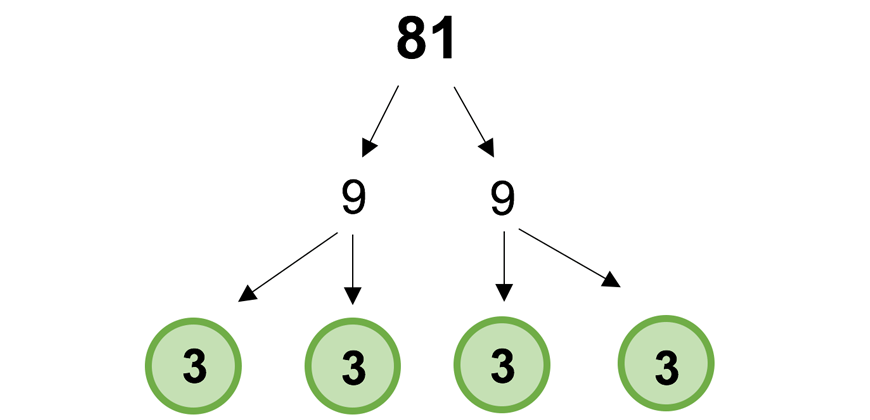We can therefore re-write $$81$$ as $$3^4$$.

\begin{align*}
\color{red}{81} &= 3^{2x} \\
\color{red}{3^4} &= 3^{2x}
\end{align*}

Notice that both sides of the equation are powers in terms of $$3$$.

Since both sides are equal, we can also say the powers on both sides must be equal.

\begin{align*}
3^{\color{lightblue}{4}} &= 3^{\color{lightblue}{2x}}\\
\color{lightblue}{4} &= \color{lightblue}{2x}\\
&2 = x
\end{align*}

## Summary

• When multiplying or dividing powers of the same base, the following index laws should be remembered:
• $$x^m \times x^n =x^{m+n}$$
• $$x^m \div x^x = x^{m-n}$$
• $$(x^m)^n = x^{mn}$$
• When multiplying powers of different bases but the same power, the distributive law applies:
• $$x^m \times y^m = (x \times y)^m$$
• When working with non-positive and non-integer powers, the following results should be remembered:
• $$x^0 = 1$$
• $$x^{-n} = \frac{1}{x^n}$$
• $$x^{\frac{1}{n}} = \sqrt[n]{x}$$
• $$x^{\frac{m}{n}} = \sqrt[n]{x^m}$$

## Get more indices practice now## Checkpoint

1. Express $$240$$ in index form by creating a factor tree.

2. By first simplifying the indices, find the value of:

$$3^2 \times 2^2 \div 2^5$$

$$x \times y \times (x + y)^3 \times 5x^2$$

4. Evaluate the following:

$$\frac{10^4}{5^4}$$

5. Find the value of the following expression:

$$8^{\frac{2}{3}}$$

6. Using the index laws, simplify the following expression. Leave your answer in index form.

$$\frac{3^{17} \div 3^2}{(3^4 \times 3^2)^2}$$

7. Simplify the following expression, leaving in index form.

$$7 \big{(} 2^{\frac{1}{3}} \big{)}$$

8. Is the following equation true or false?

$$4 \times 3^6 \div ({3^2})^2 – 3^3 = 9$$

9. Find the value of the following expression:

$$(3^{17} \div 3^{15} + 2^{18} \div 2^{14} \times 5 – 7)^0$$

10. A number is a perfect square if, when we write it as a product of its prime factors, all the powers are divisible by $$2$$. By drawing a factor tree, determine whether $$196$$ is a perfect square.

## Solutions

1. First, we must draw a factor tree for $$240$$.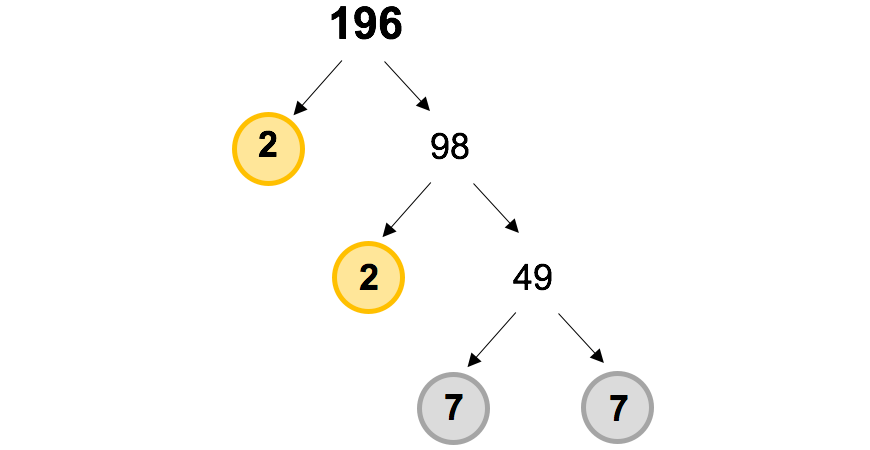Thus $$240 = 2^4 \times 3 \times 5$$

2. When approaching this question, be careful to only apply your index laws to indices with the same base.

\begin{align*}
3^2 \times 2^{2} \div 2^{5} &= 3^{2} 2^{2-5} \\
&= 3^{2} \times 2^{-3} \\
&= 3^{2} \times \frac{1}{2^{2}}\\
&= 9 \times \frac{1}{8} \\
&= \frac{9}{8}
\end{align*}

A common mistake students make in this type of question is subtracting the wrong power. Remember to subtract the power being divided.

3. Using the distributive law, we can expand the brackets.

\begin{align*}
x \times y \times \color{lightblue}{(x \times y)^3} \times 5x^2 = x \times y \times \color{lightblue}{x^3 \times y^3} \times 5x^2
\end{align*}

We can then simplify by multiplying like terms.

\begin{align*}
\color{goldenrod}{x} x \color{green}{y} \times \color{goldenrod}{x^3} \times \color{green}{y^3} \times 5\color{goldenrod}{x^2} &= 5 \times \color{goldenrod}{x^{1+3+2}} \times \color{green}{y^{1+3}} \\
&= 5x^6y^4
\end{align*}

4. Since both indices have the same power, we can use the distributive law to simplify this expression.

\begin{align*}
\frac{10^4}{5^4} &= \big{(} \frac{10}{5} \big{)}^4 \\
&= 2^4 \\
&= 16
\end{align*}

5. Recall that fractional indices can be re-written as roots.

\begin{align*}
8^{\frac{2}{3}} &= \sqrt{8^2}\\
&= \sqrt{64}\\
&= 4
\end{align*}

6. Using BODMAS, we first simplify the expression inside the brackets.

\begin{align*}
\frac{3^{17} \div 3^2}{(3^4 \times 3^2)^2} &= \frac{3^{17} \div 3^2}{(3^6)^2}\\
&= \frac{3^{17-2}}{3^{6 \times 2}} \\
&= \frac{3^{15}}{3^{12}}\\
&= 3^{15-12}\\
&= 3^3
\end{align*}

7. For this question, be careful not to raise $$7$$ to the power of $$9$$, as it is not inside the brackets.

\begin{align*}
7 \big{(} 2^{\frac{1}{3}} \big{)}^9 &= 7 \times 2^{\frac{1}{3} \times9}\\
&= 7 \times 2^3
\end{align*}

8. Starting from the left-hand side, we must use BODMAS to simplify this expression.

\begin{align*}
LHS &= 4 \times 3^6 \div (3^2)^2 – 3^3\\
&= 4 \times 3^6 \div 3^{2 \times2} – 3^3 \\
&= 4 \times 3^6 \div 3^4 – 3^3
\end{align*}

Be careful not to combine $$3^6 \div 3^4$$ and $$3^3$$, since they are separate terms.

\begin{align*}
&= 4 \times 3^{6-4} – 3^3 \\
&= 4 \times 3^2 – 3^3 \\
&=4 \times 9 – 37 \\
&= 36 – 27 \\
&= 9 \\
&= RHS
\end{align*}

Therefore this equation is TRUE.

9. All numbers taken to the power of $$0$$ are equal to $$1$$.

Therefore:

\begin{align*}
(3^{17} \div 3^{15} + 2^{18} \div 2^{14} \times – 7)^0 = 1
\end{align*}

10. First, we must draw a factor tree for $$196$$.Therefore, we can say $$196 = 2^2 \times 7^2$$.
Since all the powers are even, $$196$$ must be a perfect square.© Matrix Education and www.matrix.edu.au, 2021. Unauthorised use and/or duplication of this material without express and written permission from this site’s author and/or owner is strictly prohibited. Excerpts and links may be used, provided that full and clear credit is given to Matrix Education and www.matrix.edu.au with appropriate and specific direction to the original content.